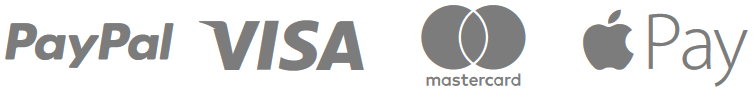25% de réduction sur le deuxième DreamyCare™ acheté

34,99€29,99€

LIMITED OFFER

• LOW IN STOCK

Quantity

SINGLE PACKBUY 1, GET 2ND 30% OFFBUY 2, GET 3RD 70% OFF
`[]`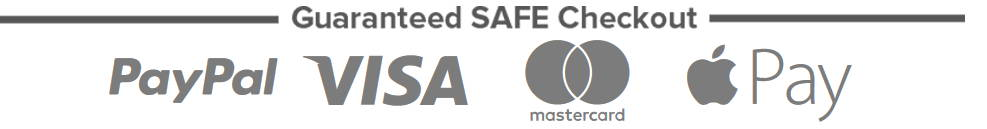\$( document ).ready(function() {function randomIntFromInterval(min, max) {return Math.floor(Math.random() * (max - min + 1) + min);}// Settings are herevar total_items = 50;var d = new Date();var min_items_left = 12;var max_items_left = 20;var remaining_items = randomIntFromInterval(min_items_left, max_items_left);var min_of_remaining_items = 2;var decrease_after = 0.27;var decrease_after_first_item = 0.23;(function(\$){\$.fn.progressbar=function(){var a="

Dépêchez-vous : seulement "+remaining_items+" en stock.

"+"
";this.addClass('items-count');this.html(a+this.html());updateMeter(this);var b=this;setTimeout(function(){remaining_items--;if(remaining_itemsb){c=e}function frame(){if(e>b){c--}else{c++}a.css('width',c+'%');if(c==b||c<=0||c>=100)clearInterval(f)}var f=setInterval(frame,40)} jQuery.noConflict()(function(\$){\$(document).ready(function(){\$("#progress_bar").progressbar();var tag="ctdn-12-12".match(/\d+/g);var hour=14;var theDaysBox=\$("#numdays");var theHoursBox=\$("#numhours2");var theMinsBox=\$("#nummins2");var theSecsBox=\$("#numsecs2");var d=new Date();var n=d.getDay();var date=1;var gg=0;var hh=0;var ii=0;var nsec=59; var nmin=19; var nhrs=0; var ndat=date-1;if(ndat<0){var mmon=d.getMonth();ndat=30+date-d.getDate()-ii}theSecsBox.html(nsec);theMinsBox.html(nmin);theHoursBox.html(nhrs);theDaysBox.html(ndat);var refreshId=setInterval(function(){var e=theSecsBox.text();var a=theMinsBox.text();var c=theHoursBox.text();var b=theDaysBox.text();if(e==0&&a==0&&c==0&&b==0){}else{if(e==0&&a==0&&c==0){theDaysBox.html(b-1);theHoursBox.html("0");theMinsBox.html("00");theSecsBox.html("59")}else{if(e==0&&a==0){theHoursBox.html(c-1);theMinsBox.html("59");theSecsBox.html("59")}else{if(e==0){theMinsBox.html(a-1);theSecsBox.html("59")}else{theSecsBox.html(e-1)}}}}},1000);});});});

DETAILS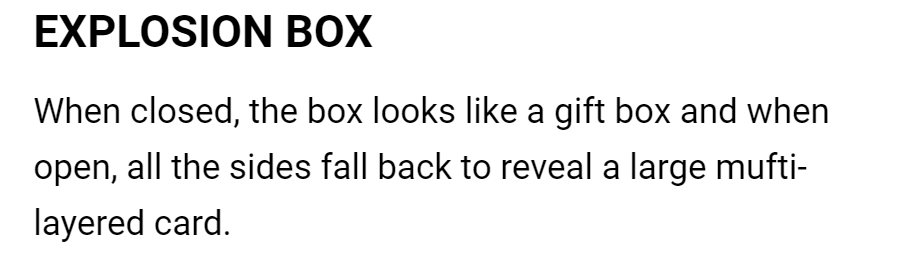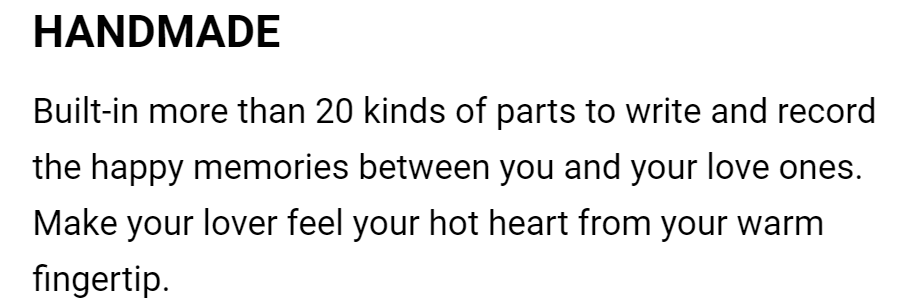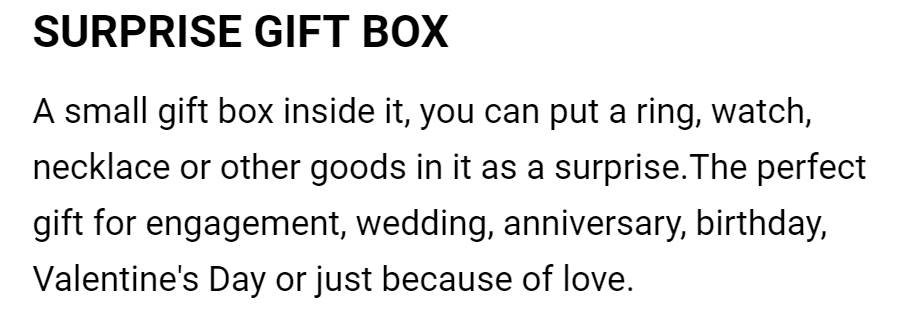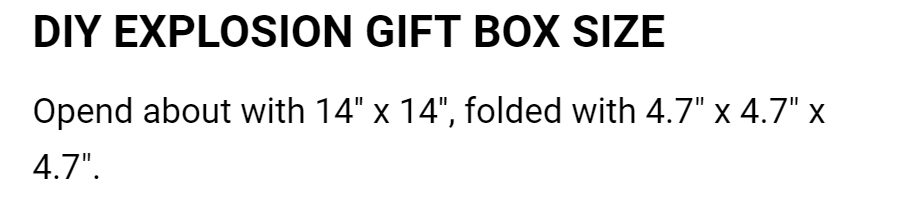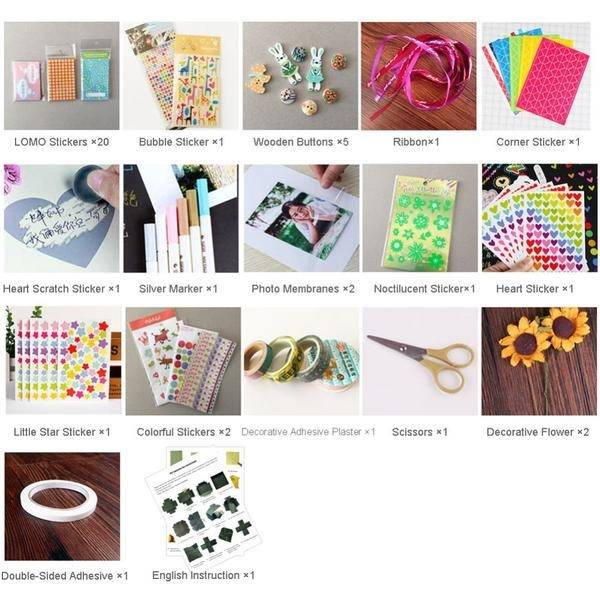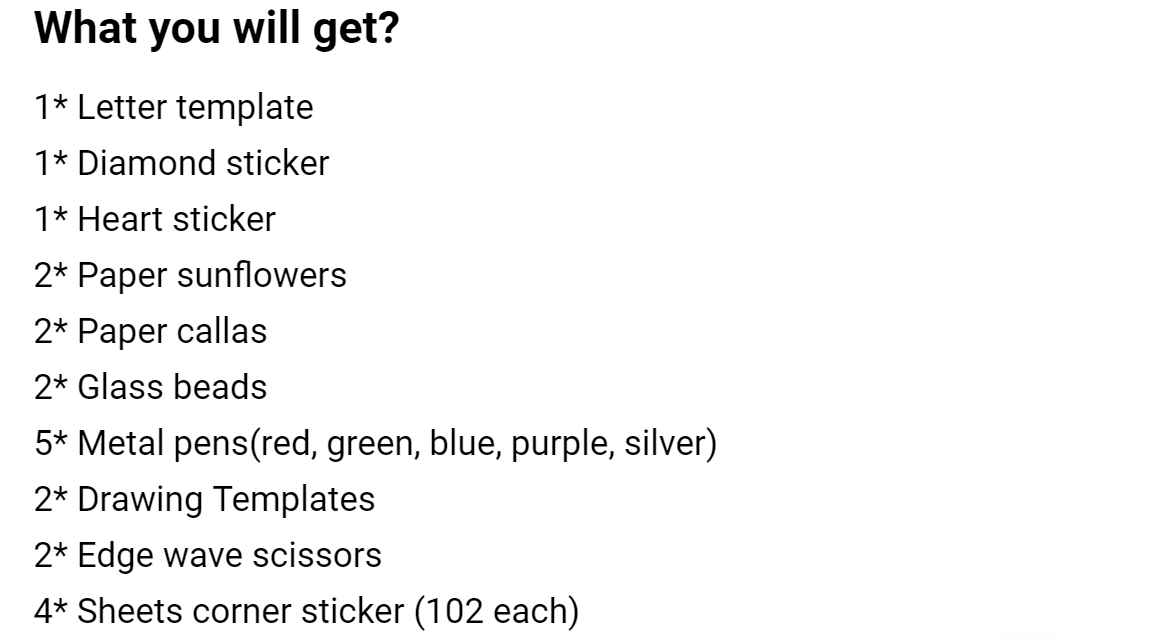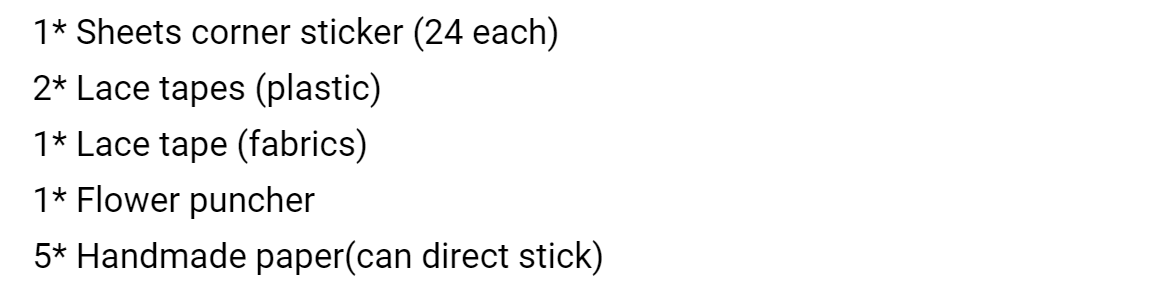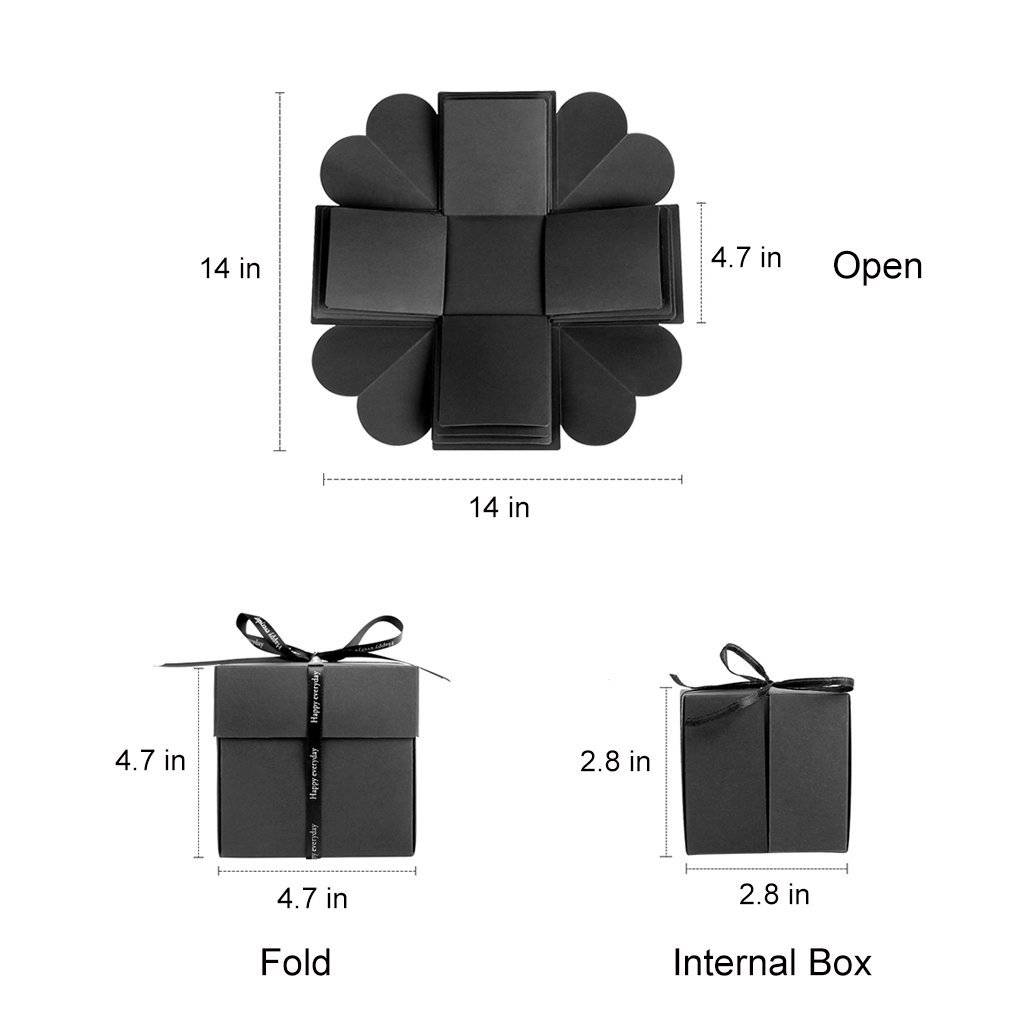34,99€29,99€34,99€29,99€We're confident we sell the very best product to the best price, and we want you to share our confidence! That's why we back every sale with a 90-day money back guarantee.

If you don't like the product, if it just doesn't work for you, return it. Within 90 days of receipt, products purchased from us may be returned for any reason. (We don't refund shipping costs unless items are defective).

CONNECT WITH US